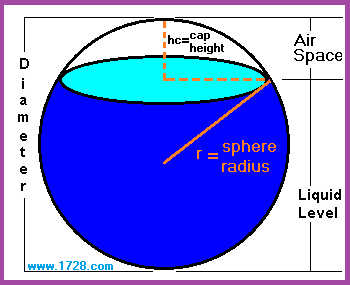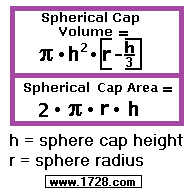Partially Filled Sphere Calculator
It calculates volume when you input radius, diameter, circumference, area or volume.
Scroll Down For Instructions>>>>>
>>>>>

or

 For ease of calculating, we chose 1) liquid level and 2) tank diameter as the data items required for input. If you only know cap height and diameter, just remember this simple relationship: Liquid Level = Diameter - Cap Height. If you need a calculator that will accept other input units, then click here. The total volume of a partially-filled spherical tank equals total sphere volume minus spherical cap volume. To see other formulas for a partially-filled spherical tank, click here. Of course you really don't need those formulas because this calculator does all the work for you.

Significant Figures >>>
Numbers are displayed in scientific notation with the amount of significant figures you specify. For easier readability, numbers between .001 and 1,000 will not be in scientific notation but will still have the same precision.
You may change the number of significant figures displayed by changing the number in the box above.
Most browsers, will display the answers properly but if you are seeing no answers at all, enter a zero in the box above, which will eliminate all formatting but at least you will see the answers.